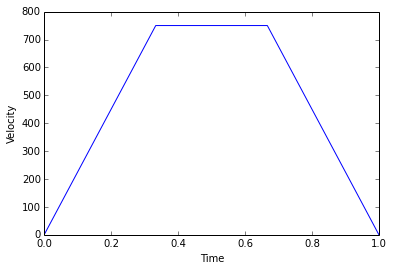# Chapter 2 : Kinematics of Motion¶

## Example 2.1 Page No: 13¶

In :
import math

# Variables:
u1 = 0
v1 = 72.*1000./3600 			#m/s
s1 = 500. 			                #m

# Solution:
# Calculating the initial acceleration of the car
a1 = (v1**2-u1**2)/(2*s1) 			#m/s**2
#Calculating time taken by the car to attain the speed
t1 = (v1-u1)/a1 			#seconds
#Parameters for the second case
u2 = v1
v2 = 90.*1000/3600 			#m/s
t2 = 10.         			#seconds

#Calculating the acceleration for the second case
a2 = (v2-u2)/t2 			#m/s**2
#Calculating the distance moved by the car in the second case
s2 = (u2*t2)+(a2/2*t2**2)
#Parameters for the third case
u3 = v2
v3 = 0 			#m/s
t3 = 5 			#seconds
#Calculating the distance moved by the car
s3 = (u3+v3)*t3/2 			#m

#Results:
print " The acceleration of the car, a  =  %.1f m/s**2. "%(a1)
print " The car takes t  =  %d s to attain the speed."%(t1)
print " The acceleration of the car in the second case, a  =  %.1f m/s**2."%(a2)
print " The distance moved by the cars  =  %d m."%(s2)
print " The distance travelled by the car during braking, s  =  %.1f m."%(s3)

 The acceleration of the car, a  =  0.4 m/s**2.
The car takes t  =  50 s to attain the speed.
The acceleration of the car in the second case, a  =  0.5 m/s**2.
The distance moved by the cars  =  225 m.
The distance travelled by the car during braking, s  =  62.5 m.


## Example 2.2 Page no : 14¶

In :
# variables
t = 1.           # second
v = 6.25         # m/s

# calculations and results
C1 = v - 0.25 +t -5

# when t = 2
t = 2.
v = t**4/4 - t**3 + 5*t + 2
print "Velocity at t=2 seconds, V = %.f m/s"%v

# when t = 1 seconds and s = 8.30 m.
t = 1.
s = 8.30
C2 = s - 1./20 + 1./4 - 5./2 - 2
t = 2.       # seconds
s = t**5/20 - t**4/4 + 5*t**2/2 + 2*t + 4
print "Displacement = %.1f m"%s

Velocity at t=2 seconds, V = 8 m/s
Displacement = 15.6 m


## Example 2.3 Page No: 15¶

In :
import math

# Variables:
#Initial parameters
v0 = 100. 			#kmph
t0 = 0
#Parameters at the end of 40 seconds
v1 = 90./100*v0 			#kmph
t1 = 40.         			#seconds

#Solution:
#The acceleration is given by
#a = (-dv/dt) = k*v
#Integrating
#we get ln(v) = -k*t+C
#Calculating the constant of integration
def f3(v):
return 1./v

#Calculating the constant of proportionality
k = (C-2.3*math.log10(90))/40
#Time after 120 seconds
t2 = 120. 			#seconds
#Calculating the velocity after 120 seconds
v120 = 10**((-k*t2+C)/2.29)

#Results:
print " The velocity at the end of 120 seconds =  %.1f kmph."%(v120)

 The velocity at the end of 120 seconds =  73.5 kmph.


## Example 2.5 Page No: 17¶

In :
%matplotlib inline

import math
from matplotlib.pyplot import *

# Variables:
s = 500.        #mm
s1 = 125.       #mm
s2 = 250.       #mm
s3 = 125. 		#mm
t = 1. 			#second

#Solution:
#Matrices for the velocity vs. time graph
V = [0 ,750.,750.,0] 			#The velocity matrix
T = [0,1./3,2./3,1] 			#The time matrix
plot(T,V)
xlabel("Time")
ylabel("Velocity")
#Calculating the time of uniform acceleration

#Equating the time taken to complete the stroke to 1 second
v = (125/(1./2)+250/1+125/(1./2))/1 			#mm/s

#Results:
show()
print " The maximum cutting speed  v  =  %d mm/s."%(v)

Populating the interactive namespace from numpy and matplotlibThe maximum cutting speed  v  =  750 mm/s.


## Example 2.6 Page No: 19¶

In :
# Variables:
N0 = 0
N = 2000. 			#rpm
t = 20. 			#seconds

#Solution:
#Calculating the angular velocities
omega0 = 0
#Calculating the angular acceleration
#Calculating the angular distance moved by the wheel during 2000 rpm
#Calculating the number of revolutions made by the wheel
n = theta/(2*math.pi)

#Results:
print " The angular acceleration of the wheel, alpha  =  %.3f rad/s**2."%(alpha)
print " The wheel makes n  =  %.1f revolutions."%(n)

 The angular acceleration of the wheel, alpha  =  10.472 rad/s**2.
The wheel makes n  =  333.3 revolutions.


## Example 2.7 Page No: 21¶

In :
import math

# Variables:
r = 1.5 			#m
N0 = 1200.
N = 1500. 			#rpm
t = 5. 			#seconds

#Solution:
#Calculating the angular velocities
omega0 = 2*math.pi*N0/60
#Calculating the linear velocity at the beginning
v0 = r*omega0 			#m/s
#Calculating the linear velocity at the end of 5 seconds
v5 = r*omega 			#m/s
#Calculating the angular acceleration
#Calculating the math.tangential acceleration after 5 seconds
TangentialAcceleration = alpha*(r/2) 			#m/s**2
#Calculating the radial acceleration after 5 seconds

 The linear velocity at the beginning, v0  =  188.5 m/s.